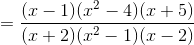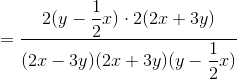# Solutions to Questions Multiply, Divide, Simplify Rational Expressions

Detailed solutions to questions in How to multiply, divide and simplify rational expressions are presented.

 Rules of multiplication, division and simplification of rational expressions? We multiply two rational expressions by multiplying their numerators and denominators as follows: 1)We divide two rational expressions by multiplying the first by the reciprocal of the second as follows: 2)Simplify the rational expressions a)Solution: The division of two rational expressions is done by multiplying the first by the reciprocal of the second as follows (see division rule above). HenceMultiply numerators and denominators (multiplication rule).Factor the terms 6x-9 included in the denominator as follows: 6x - 9 = 3(2x - 3) and use the factored form in the rational expressionsimplifyb)Solution: Apply the multiplication rule (see above)Factor terms in the numerator and the denominator: 10 x + 10 = 10(x + 1) ; 2 x + 2 = 2(x + 1) ; 4 x - 10 = 2(2x - 5) and use in factored formSimplify if possiblec)Solution: The division of two rational expressions is done by multiplying the first rational expression by the reciprocal of the second rational expression as follows (see division rule above). HenceMultiply numerators and denominators (multiplication rule) but do not expand as we might be able to simplifty.factor terms in the numerator and denominator if possible. 2 x 2 - 7 x - 15 = (2x + 3)(x - 5) ; x 2 - 1 = (x - 1)(x + 1) x 2 + 3 x - 4 = (x + 4)(x - 1) ; x 2 + x - 30 = (x + 6)(x - 5) and use in rational expressionand simplify.d)Solution: We have the multiplication of two rational expressions inside the parentheses and we then apply multiplication rule. We also have a division by a rational expression which is done by multiplying by the reciprocal. HenceMultiply numerators and denominators (multiplication rule) but do not expand as we might be able to simplify.Factor terms in numerator and denominator if possible and use in rational expression x 2 - 4 = (x - 2)(x + 2) and x 2 - 1 = (x - 1)(x + 1)Simplifye)Solution: The division of two rational expressions is done by multiplying the first rational expression by the reciprocal of the second rational expression as follows (see division rule above). HenceMultiply numerators and denominators (multiplication rule) but do not expand.factor term x 3 - 27 in the numerator and use it. x 3 - 27 = (x - 3)(x 2 + 3 x + 9) and use in rational expressionand simplify.f)Solution: Apply the multiplication rule (see above)Factor terms in the numerator and the denominator: 2 y - x = 2 (y - (1/2) x) ; 4 x + 6 y = 2(2 x + 3) ; 4 x 2 - 9 y 2 = (2x - 3y)(2x + 3y) and use in factored formSimplify if possible